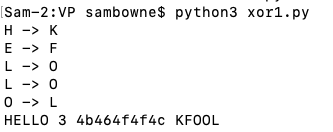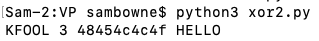VP 310: XOR (65 pts)

Purpose

Encrypt data with XOR, and break XOR encryption.

Purpose

Encrypt and decrypt files using XOR in Python.

Understanding XOR

Exclusive OR (XOR) is a fundamental mathematical operation used in many encryption algorithms.

XOR operates on one bit at a time, with these results:

0 XOR 0 = 0
0 XOR 1 = 1
1 XOR 0 = 1
1 XOR 1 = 0
For our purposes, we'll use the Python ^ operator, which acts on a whole byte at a time.

Characters are ASCII-encoded, like this:

A is 01000001
B is 01000010
C is 01000011
...
A whole table of ASCII values is here:

Consider A^B:

A is 01000001
B is 01000010
A^B= 00000011
That is character 3, an unprintable end-of-text mark.

However, A^s is printable:

A is 01000001
s is 01110011
A^B= 00110010
The result is the hexadecimal value 0x32, or the numeral 2.

XOR in Python

Make a program containing this code:
plaintext = "HELLO"
key = 3
ciphertext = ""
for char in plaintext:
enc_num = ord(char) ^ key
enc_char = chr(enc_num)
print(char, "->", enc_char)
ciphertext += enc_char
print(plaintext, key, ciphertext.encode().hex(), ciphertext)
Run the program. It shows how each character is encoded. The output is readable in this case, but often it isn't, so printing it in hex format is best.Encrypting a Second Time

Make a program containing this code:
plaintext = "KFOOL"
key = 3
ciphertext = ""
for char in plaintext:
enc_num = ord(char) ^ key
enc_char = chr(enc_num)
# print(char, "->", enc_char)
ciphertext += enc_char
print(plaintext, key, ciphertext.encode().hex(), ciphertext)
Run the program. As shown below, encrypting ciphertext again with the same key reverses the process, returning the original plaintext.Flag VP 310.1: Encrypt Text (5 pts)

Encrypt this text with a key of 7:
WALDO
The flag is the ciphertext, as a five-letter word.

Flag VP 310.2: Decrypt Text With the Key (5 pts)

Decrypt this text with a key of 19:
\QUF@PRGVW
The flag is the ciphertext, as a five-letter word.

Flag VP 310.3: Decrypt Text Without the Key (10 pts)

Decrypt this ciphertext, which is expressed in hexadecimal encoding:
c39bc3b1c3bcc3bac2a7c2bdc39fc38fc388c389c398c39bc392c38fc39ec398
The key is between 0 and 255.

The flag is in the plaintext, as a readable word.

Flag VP 310.4: Decrypt an Image Without the Key (15 pts)

VP310-4
Decrypt it to find a PNG image. View that image to see the flag.

The key is a single byte between 0 and 255.

Flag VP 310.5: Two-Byte Key (15 pts)

VP310-5
Decrypt it to find a JPEG image. View that image to see the flag.

The key is two bytes long, so encryption takes place sixteen bits at a time.